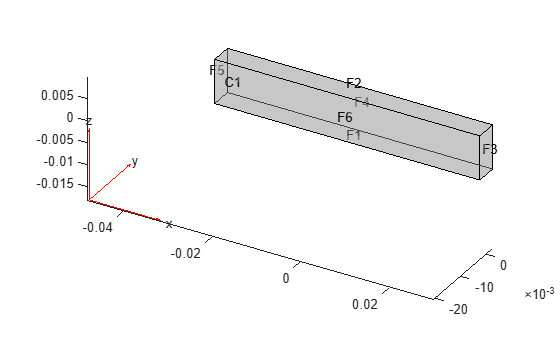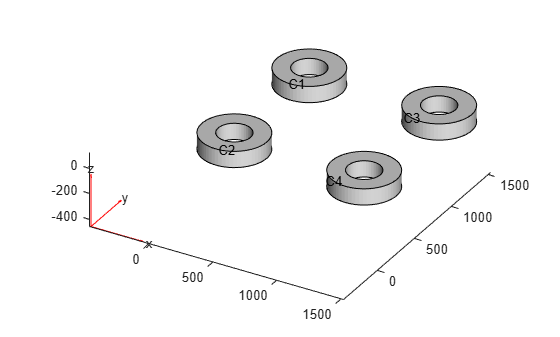# cellIC

Initial conditions on geometry cell

Since R2023a

## Description

A `cellIC` object specifies the type of an initial condition on a cell of a geometry. An `femodel` object contains an array of `cellIC` objects in its `CellIC` property.

`cellIC` applies these rules for initial condition assignments:

• You can use a geometric assignment to associate the initial condition with the specified geometric regions or the entire geometry.

• You must apply a results-based assignment to all geometric regions because the `results` object contains information about the entire geometry.

• For separate assignments to a geometric region (or the entire geometry) and the boundaries of that region, the solver uses the specified assignment on the region and chooses the assignment on the boundary as follows. The solver gives an `EdgeIC` assignment precedence over a `FaceIC` assignment, even if you specify a `FaceIC` assignment after an `EdgeIC` assignment. The assignments in order of precedence are `VertexIC` (highest precedence), `EdgeIC`, `FaceIC`, and `CellIC` (lowest precedence).

## Creation

### Syntax

``model.CellIC(CellID) = cellIC(Name=Value)``
``model.CellIC = cellIC(Name=Value)``

### Description

example

````model.CellIC(CellID) = cellIC(Name=Value)` creates a `cellIC` object and sets properties using one or more name-value arguments. This syntax assigns the specified structural, thermal, or electromagnetic initial condition to the specified cells of the geometry stored in the `femodel` object `model`. For example, `model.CellIC([1 2]) = cellIC(Temperature=25)` specifies the temperature on cells 1 and 2.```

example

````model.CellIC = cellIC(Name=Value)` assigns the specified initial condition to the entire geometry. For example, ```model.CellIC = cellIC(Temperature = 25)``` specifies the initial temperature on all cells of the geometry.```

### Input Arguments

expand all

Cell IDs, specified as a vector of positive integers. Find the cell IDs using `pdegplot` with the `CellLabels` value set to `"on"`.

Data Types: `double`

## Properties

expand all

Initial displacement, specified as a numeric vector of three elements, function handle, `StaticStructuralResults` object, or `TransientStructuralResults` object created by using `solve`. The elements of the numeric vector represent the x-, y-, and z-components of initial displacement.

Use a function handle to specify spatially varying initial displacement. The function must return a three-row matrix. Each column of the matrix corresponds to the initial displacement at the coordinates provided by the solver. For details, see Nonconstant Parameters of Finite Element Model.

When you specify initial displacement as a `StaticStructuralResults` or `TransientStructuralResults` object, `cellIC` applies the results to the entire geometry. For `TransientStructuralResults`, you can access results for a particular time-step by using the `filterByIndex` function.

Initial velocity, specified as a numeric vector of three elements, function handle, `StaticStructuralResults` object, or `TransientStructuralResults` object created by using `solve`. The elements of the numeric vector represent the x-, y-, and z-components of initial velocity.

Use a function handle to specify spatially varying initial velocity. The function must return a three-row matrix. Each column of the matrix corresponds to the initial velocity at the coordinates provided by the solver. For details, see Nonconstant Parameters of Finite Element Model.

When you specify initial velocity as a `StaticStructuralResults` or `TransientStructuralResults` object, `cellIC` applies the results to the entire geometry. For `TransientStructuralResults`, you can access results for a particular time-step by using the `filterByIndex` function.

Initial temperature or initial guess for temperature, specified as a real number, function handle, `SteadyStateThermalResults` object, or `TransientThermalResults` object. Use a function handle to specify spatially varying initial temperature. For details, see Nonconstant Parameters of Finite Element Model.

When you specify initial temperature as a `SteadyStateThermalResults` or `TransientThermalResults` object, `cellIC` applies the results to the entire geometry. For `TransientThermalResults`, you can access results for a particular time-step by using the `filterByIndex` function.

Initial guess for magnetic potential in a nonlinear magnetostatic problem, specified as a column vector of three elements, a function handle, or a `MagnetostaticResults` object. Use a function handle to specify spatially varying initial magnetic potential. For details, see Nonconstant Parameters of Finite Element Model.

Initial flux density, specified as a positive number or a function handle. Use a function handle to specify an initial flux density that depends on the coordinates, magnetic potential and its gradients, and the norm of magnetic flux density. For details, see Nonconstant Parameters of Finite Element Model.

If a relative permeability, current density, or magnetization for the model depend on the magnetic potential or its gradients (`state.u`, `state.ux`, and so on), then initial conditions must not depend on the magnetic flux density (`state.NormFluxDensity`).

## Examples

collapse all

Specify initial temperature on a cell for an `femodel` object representing a transient thermal problem.

Create and plot a geometry that consists of two nested cylinders.

```gm = multicylinder([0.01,0.015],0.05); gm = rotate(gm,90,[0 0 0],[0 1 0]); pdegplot(gm,CellLabels="on",FaceAlpha=0.4);```Create an `femodel` object for solving a transient thermal problem, and assign the geometry to the model.

```model = femodel(AnalysisType="thermalTransient", ... Geometry=gm);```

Specify the initial temperature for the inner cylinder.

```model.CellIC(1) = cellIC(Temperature=100); model.CellIC```
```ans = 1x2 cellIC array Properties for analysis type: thermalTransient Index Temperature 1 100 2 [] Show all properties ```

Specify initial displacement on a beam geometry for an `femodel` object representing a transient structural problem.

Create and plot a beam geometry.

```gm = multicuboid(0.06,0.005,0.01); pdegplot(gm,FaceLabels="on", ... CellLabels="on", ... FaceAlpha=0.4);```Create an `femodel` object for solving a static structural problem, and assign the geometry to the model.

```model = femodel(AnalysisType="structuralStatic", ... Geometry=gm);```

Specify Young's modulus, Poisson's ratio, and the mass density.

```model.MaterialProperties = materialProperties(YoungsModulus=210e3, ... PoissonsRatio=0.3, ... MassDensity=2.7e-6);```

Apply the boundary condition and static load.

```model.FaceBC(5) = faceBC(Constraint="fixed"); model.FaceLoad(3) = faceLoad(SurfaceTraction=[0 1e6 0]);```

Generate a mesh and solve the model.

```model = generateMesh(model,Hmax=0.02); Rstatic = solve(model);```

Change the analysis type of the model to structural transient.

`model.AnalysisType = "structuralTransient";`

Specify the initial condition using the static solution.

```model.CellIC = cellIC(Displacement=Rstatic); model.CellIC```
```ans = 1x1 cellIC array Properties for analysis type: structuralTransient Index Displacement Velocity Temperature 1 [1x1 pde.StaticStructuralResults] [] [] Show all properties ```

Specify initial magnetic potential for an `femodel` object representing a nonlinear magnetostatic problem.

Create an `femodel` object for solving a magnetostatic problem, and assign a geometry consisting of four hollow cylinders to the model.

```model = femodel(AnalysisType="magnetostatic", ... Geometry="DampingMounts.stl");```

Plot the geometry with the cell labels.

`pdegplot(model.Geometry,CellLabels="on");`Specify the initial magnetic potential for the entire geometry.

```model.CellIC = cellIC(MagneticVectorPotential=[0.1 0.1 0]); model.CellIC```
```ans = 1x4 cellIC array Properties for analysis type: magnetostatic Index MagneticVectorPotential NormFluxDensity 1 [0.1000 0.1000 0] [] 2 [0.1000 0.1000 0] [] 3 [0.1000 0.1000 0] [] 4 [0.1000 0.1000 0] [] Show all properties ```

Specify the initial magnetic potential for cell 1.

```model.CellIC(1) = cellIC(MagneticVectorPotential=[0.2 0.1 0]); model.CellIC```
```ans = 1x4 cellIC array Properties for analysis type: magnetostatic Index MagneticVectorPotential NormFluxDensity 1 [0.2000 0.1000 0] [] 2 [0.1000 0.1000 0] [] 3 [0.1000 0.1000 0] [] 4 [0.1000 0.1000 0] [] Show all properties ```

## Version History

Introduced in R2023a